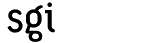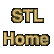# lexicographical_compare_3wayCategory: algorithms Component type: function

### Prototype

```template <class InputIterator1, class InputIterator2>
int lexicographical_compare_3way(InputIterator1 first1, InputIterator1 last1,
InputIterator2 first2, InputIterator2 last2);
```

### Description

Lexicographical_compare_3way is essentially a generalization of the function strcmp from the standard C library: it returns a negative number if the range [first1, last1) is lexicographically less than the range [first2, last2), a positive number if [first2, last2) is lexicographically less than [first1, last1), and zero if neither range is lexicographically less than the other. 

As with lexicographical_compare, lexicographical comparison means "dictionary" (element-by-element) ordering. That is, lexicographical_compare_3way returns a negative number if *first1 is less than *first2, and a positive number if *first1 is greater than *first2. If the two first elements are equivalent  then lexicographical_compare_3way compares the two second elements, and so on. Lexicographical_compare_3way returns 0 only if the two ranges [first1, last1) and [first2, last2) have the same length and if every element in the first range is equivalent to its corresponding element in the second.

### Definition

Defined in the standard header algorithm, and in the nonstandard backward-compatibility header algo.h. This function is an SGI extension; it is not part of the C++ standard.

### Requirements on types

• InputIterator1 is a model of Input Iterator.
• InputIterator2 is a model of Input Iterator.
• InputIterator1's value type is a model of LessThan Comparable.
• InputIterator2's value type is a model of LessThan Comparable.
• If v1 is an object of InputIterator1's value type and v2 is an object of InputIterator2's value type, then both v1 < v2 and v2 < v1 are defined.
• Operator< is a strict weak ordering, as defined in the LessThan Comparable requirements.

### Preconditions

• [first1, last1) is a valid range.
• [first2, last2) is a valid range.

### Complexity

Linear. At most 2 * min(last1 - first1, last2 - first2) comparisons.

### Example

```int main()
{
int A1[] = {3, 1, 4, 2, 8, 5, 7};
int A2[] = {3, 1, 4, 1, 5, 9, 3};
int A3[] = {1, 2, 3, 4};
int A4[] = {1, 2, 3, 4, 5};

const int N1 = sizeof(A1) / sizeof(int);
const int N2 = sizeof(A2) / sizeof(int);
const int N3 = sizeof(A3) / sizeof(int);
const int N4 = sizeof(A4) / sizeof(int);

int C12 = lexicographical_compare_3way(A1, A1 + N1, A2, A2 + N2);
int C34 = lexicographical_compare_3way(A3, A3 + N3, A4, A4 + N4);

cout << "A1[] and A2[]: " << C12 << endl;
cout << "A3[] and A4[]: " << C34 << endl;
}
```

### Notes

 Lexicographical_compare_3way is almost, but not quite, redundant: the call lexicographical_compare_3way(f1,l1, f2,l2) could be written as lexicographical_compare(f1,l1, f2,l2) ? -1 : (lexicographical_compare(f2,l2, f1,l1) ? 1 : 0). The single call to lexicographical_compare_3way, however, is much faster than the two calls to lexicographical_compare.

 "Equivalent", not "equal", because two equivalent elements (that is, two elements with the property that neither one is less than the other) are not necessarily equal. Operator< is required to induce a strict weak ordering, not necessarily a total ordering. See the LessThan Comparable requirements for a discussion.